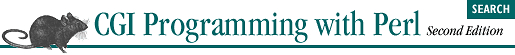home | O'Reilly's CD bookshelfs | FreeBSD | Linux | Cisco | Cisco Exam## 4.3. Decoding Form Input

```sub parse_form_data {
my %form_data;
my \$name_value;
my @name_value_pairs = split /&/, \$ENV{QUERY_STRING};

if ( \$ENV{REQUEST_METHOD} eq 'POST' ) {
my \$query = "";
read( STDIN, \$query, \$ENV{CONTENT_LENGTH} ) == \$ENV{CONTENT_LENGTH}
or return undef;
push @name_value_pairs, split /&/, \$query;
}

foreach \$name_value ( @name_value_pairs ) {
my( \$name, \$value ) = split /=/, \$name_value;

\$name =~ tr/+/ /;
\$name =~ s/%([\da-f][\da-f])/chr( hex(\$1) )/egi;

\$value = "" unless defined \$value;
\$value =~ tr/+/ /;
\$value =~ s/%([\da-f][\da-f])/chr( hex(\$1) )/egi;

\$form_data{\$name} = \$value;
}
return %form_data;
}```
```my %query = parse_form_data(  ) or error( "Invalid request" );
my \$activity = \$query{activity};```

You may have noticed that there is a problem with our subroutine; it occurs in the hash assignment near the end of the subroutine:

`\$form_data{\$name} = \$value;`
`numbers=One&numbers=Two`

Our example earlier would save only the last value in the hash. There are a couple different ways we could solve this, but neither is ideal. First, we could convert the value of the hash into an array reference for multiple values by replacing the hash assignment with the following lines:

```if ( exists \$form_data{\$name} ) {
if ( ref \$form_data{\$name} ) {
push @{ \$form_data{\$name} }, \$value;
}
else {
\$form_data{\$name} = [ \$form_data{\$name}, \$value ];
}
else {
\$form_data{\$name} = \$value;
}```
```my %query = parse_form_data(  ) or error( "Invalid request" );
my @numbers = ref( \$query{numbers} ) ? @{ \$query{numbers} } : \$query{numbers};```

This syntax is awkward. Another approach is to store the multiple values as a single text string that is delimited by a certain character, such as a tab or "\0". This is easier to code in the subroutine:

```if ( exists \$form_data{\$name} ) {
\$form_data{\$name} .= "\t\$value";
else {
\$form_data{\$name} = \$value;
}```

It is also easier to read in the CGI script:

```my %query = parse_form_data(  ) or error( "Invalid request" );
my @numbers = split "\t", \$query{numbers};```

However, there is still a potential for corrupted data if the CGI script is not expecting multiple values.